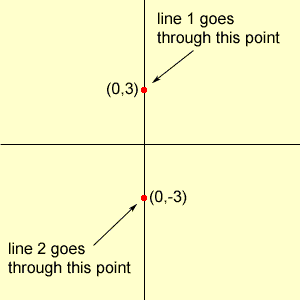Quandaries and Queries how do you solve y=1\3x+3 and y=1\3x-3 by graphing? Hi, I think it is realy important with equations of lines that you understand what the numbers mean. This makes it very easy to graph the equation and, in particular, solve the problem you have. You have two equations of the form y = mx + b where m and b are numbers. It is these two numbers, m and b, that tell you how to graph the line. m is the slope of the line The two lines that you have both have slope 1/3. b is the y-intercept. This means that the line crosses the y-axis at the point where y=b. In other words (0,b) is on the line. Your first line crosses the y-axis at (0,3) and your second line crosses the y-axis at (0,-3)What do you know about two lines with the same slope? Penny Go to Math Central